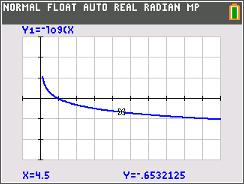••• ##### Device
• TI-84 Plus
• TI-84 Plus Silver Edition
•TI-84 Plus C Silver Edition
•TI-84 Plus CE
• ##### Report an Issue

Precalculus: Can You Hear Me Now?
by Texas Instruments#### Overview

Students will explore logarithmic equations relating to sound intensity and pH.

#### Key Steps

•Students engage in an exploration of logarithmic functions with real applications. Problem 1 involves the exploration of the relationship between the intensity of sound in W / m2 and the corresponding sound intensity level in decibels (dB). Students observe the large range of values for measured sound intensity and learn that logarithmic functions are helpful in working with data involving large ranges of possible values.

•A second, similar problem is provided as either an extension or for homework. This activity involves the pH scale and students similarly perform calculations in a spreadsheet. Given pH of a variety of common solutions, students are asked to determine the corresponding hydrogen ion concentrations.